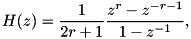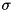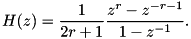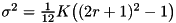A Survey of Gaussian Convolution Algorithms
Box filter Gaussian convolution

A fast low-accuracy approximation of Gaussian convolution. More...

## Detailed Description

A fast low-accuracy approximation of Gaussian convolution.

This code implements the basic iterated box filter approximation of Gaussian convolution as developed by Wells. This approach is based on the efficient recursive implementation of the box filter aswhere r is the box radius.

While box filtering is very efficient, it has the limitation that only a quantized set ofvalues can be approximated because the box radius r is integer. Extended box filter Gaussian convolution and Stacked integral images Gaussian convolution are extensions of box filtering that allowto vary continuously.

Example
buffer = (num *)malloc(sizeof(num) * N);
free(buffer);
Reference
• W.M. Wells, "Efficient synthesis of Gaussian filters by cascaded uniform filters," IEEE Transactions on Pattern Analysis and Machine Intelligence, vol. 8, no. 2, pp. 234-239, 1986. http://dx.doi.org/10.1109/TPAMI.1986.4767776

## Functions

static void box_filter (num *dest, long dest_stride, const num *src, long src_stride, long N, long r)
Perform one pass of box filtering. More...

void box_gaussian_conv (num *dest, num *buffer, const num *src, long N, long stride, num sigma, int K)
Box filtering approximation of Gaussian convolution. More...

void box_gaussian_conv_image (num *dest, num *buffer, const num *src, int width, int height, int num_channels, num sigma, int K)
Box filtering approximation of 2D Gaussian convolution. More...

## Function Documentation

 static void box_filter ( num * dest, long dest_stride, const num * src, long src_stride, long N, long r )
static

Perform one pass of box filtering.

Parameters
 dest destination array dest_stride stride between successive samples of dest src_stride src array (must be distinct from dest) src_stride stride between successive samples of src N number of samples r radius of the box filter

Performs one pass of box filtering with radius r (diameter 2r+1) according to the recursive filterNote
The computation is out-of-place, src and dest must be distinct.

Definition at line 43 of file gaussian_conv_box.c.

 void box_gaussian_conv ( num * dest_data, num * buffer_data, const num * src, long N, long stride, num sigma, int K )

Box filtering approximation of Gaussian convolution.

Parameters
 dest_data destination array buffer_data array with space for at least N samples src input, overwritten if src = dest_data N number of samples stride stride between successive samples sigma Gaussian standard deviation in pixels K number of box filter passes
Note
This routine can only approximate Gaussian convolution for a quantized set ofvalues. The input argument sigma is rounded to the closest supported value.

This routine performs iterated box filtering approximation of Gaussian convolution. Well's approximationis used to select the box filter radius.

The filtering itself is performed by calling the box_filter() function K times. Since box_filter() requires that the source and destination arrays are distinct, the iteration alternates the roles of the dest and buffer arrays. For example the iteration pseudocode for K = 4 is

    buffer <- box_filter(src)
dest   <- box_filter(buffer)
buffer <- box_filter(dest)
dest   <- box_filter(buffer)
dest   <- dest * scale


The convolution can be performed in-place by setting src = dest (the source array is overwritten with the result). However, buffer_data must be distinct from dest_data.

Definition at line 105 of file gaussian_conv_box.c.

 void box_gaussian_conv_image ( num * dest, num * buffer, const num * src, int width, int height, int num_channels, num sigma, int K )

Box filtering approximation of 2D Gaussian convolution.

Parameters
 dest destination image buffer array with at least max(width,height) samples src input, overwritten if src = dest width image width height image height num_channels number of image channels sigma Gaussian standard deviation in pixels K number of box filter passes

Similar to box_gaussian_conv(), this routine approximates 2D Gaussian convolution with box filtering.

The convolution can be performed in-place by setting src = dest (the source array is overwritten with the result). However, buffer must be distinct from dest.

Definition at line 195 of file gaussian_conv_box.c.